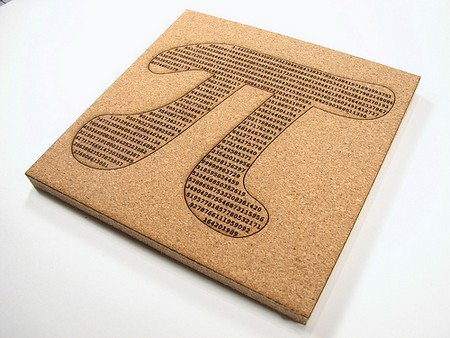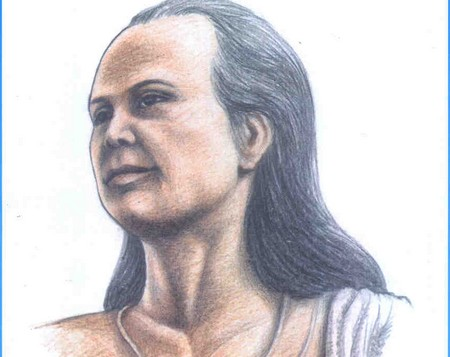Oct
3
2009

# Who Discovered Pi

Pi is a strange number. It is an irrational yet real number that is equal to 3.14159. According to Euclid, 3.14159 is the ratio of a circle’s circumference to its diameter. No matter how many decimals you bring Pi to, it never repeats a number sequence.

Earliest calculators of Pi

Pi has been known and used for thousands of years.   The ancient mathematicians of Babylon were using Pi as early as the 19th BC. Pi’s value at the time was equal to 25/8. It was pretty close to Pi’s real value by 0.5 %.
On the other hand, the ancient Egyptian scholar named Ahmes wrote the oldest value for Pi. He calculated Pi to be 256 divided by 81 or 3.160. Ahmes claimed to have found that equation in a papyrus from the Middle Kingdom.
Aryabhata was an Indian astronomer and mathematician who lived in the 5th century BC. He calculated the value of Pi as equivalent to 62832/2000, or 3.1416. He got it correct when the figure was rounded off to four decimal places. Aryabhata did not ever say he was absolutely correct. He was humble enough to say his was merely an estimate.In the far east, a Chinese mathematician calculated the value of Pi between 3.1415927 and 3.1415926. Zu Chongzhi came up with two close estimates, 355/113 and 22/7, around the 5th century.  About  that time, too, Liu Hui computed ? to be  3.141014. He believed  Zu Chongzhi and Aryabhata’s values did not much vary. Lui Hui said that  3.14 was a reliable and logical value.
Back in India, Madhava from Sangamagrama came up with the value of Pi when he changed the power series of Pi /4 into the equation. Then, he used the first 21 numbers of the sequence to solve for a logical estimate of Pi. He got it correct up to 11 decimal places. When he added a remainder term to the original number sequence, he was able to solve Pi correctly up to the 13th decimal places.

Two hundred years later, a Persian astronomer named Ghyath ad-din Jamshid Ksahani computed Pi correctly up the 9th digit in the base of 60. It was equivalent to 16 decimal digits.Biblical  references to Pi

It may sound far-fetched but the Bible mentions Pi. In 1 Kings 7:23, the Gospel says that the measurements for a round basin have a 30 cubit circumference and 10 cubit diameter. Rabbis would say that this computation is based on the circumference of the brim and the diameter from the outside across. When computed, the value would be close to 3.14.

Archimedes’ Experiment

Archimedes of Syracuse, Greece, is the widely recognized mathematician behind the correct value of Pi. He lived between 287-212 BC. He came up with the figure by studying the perimeters of a polygon with 96 sides, drawing a circle and being drawn by it in return. When he computed the average of the two values, he came up with 3.1419.
Pi is a very common figure in mathematics. What is strange is that it sometimes appears in an equation where it is not needed.

### Related Guides